# NCERT solutions for Mathematics Exemplar Class 9 chapter 6 - Lines & Angles [Latest edition]

#### Chapters## Chapter 6: Lines & Angles

Exercise 6.1Exercise 6.2Exercise 6.3Exercise 6.4
Exercise 6.1 [Pages 55 - 56]

### NCERT solutions for Mathematics Exemplar Class 9 Chapter 6 Lines & Angles Exercise 6.1 [Pages 55 - 56]

#### Choose the correct alternative:

Exercise 6.1 | Q 1 | Page 55

In figure, if AB || CD || EF, PQ || RS, ∠RQD = 25° and ∠CQP = 60°, then ∠QRS is equal to ______.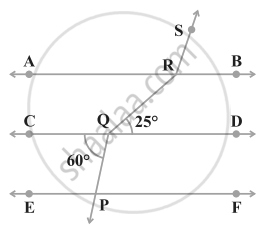• 85°

• 135°

• 145°

• 110°

Exercise 6.1 | Q 2 | Page 55

If one angle of a triangle is equal to the sum of the other two angles, then the triangle is ______.

• An isosceles triangle

• An obtuse triangle

• An equilateral triangle

• A right triangle

Exercise 6.1 | Q 3 | Page 55

An exterior angle of a triangle is 105° and its two interior opposite angles are equal. Each of these equal angles is ______.

• 37 1/2^circ

• 52 1/2^circ

• 72 1/2^circ

• 75°

Exercise 6.1 | Q 4 | Page 55

The angles of a triangle are in the ratio 5:3:7. The triangle is ______.

• An acute-angled triangle

• An obtuse-angled triangle

• A right triangle

• An isosceles triangle

Exercise 6.1 | Q 5 | Page 55

If one of the angles of a triangle is 130°, then the angle between the bisectors of the other two angles can be ______.

• 50°

• 65°

• 145°

• 155°

Exercise 6.1 | Q 6 | Page 55

In figure, POQ is a line. The value of x is ______.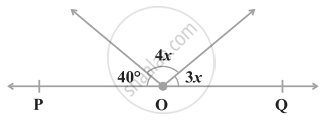• 20°

• 25°

• 30°

• 35°

Exercise 6.1 | Q 7 | Page 56

In figure, if OP||RS, ∠OPQ = 110° and ∠QRS = 130°, then ∠ PQR is equal to ______.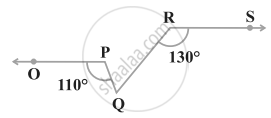• 40°

• 50°

• 60°

• 70°

Exercise 6.1 | Q 8 | Page 56

Angles of a triangle are in the ratio 2:4:3. The smallest angle of the triangle is ______.

• 60°

• 40°

• 80°

• 20°

Exercise 6.2 [Pages 56 - 57]

### NCERT solutions for Mathematics Exemplar Class 9 Chapter 6 Lines & Angles Exercise 6.2 [Pages 56 - 57]

Exercise 6.2 | Q 1 | Page 56

For what value of x + y in figure will ABC be a line? Justify your answer.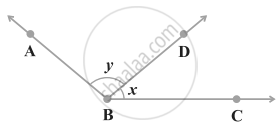Exercise 6.2 | Q 2 | Page 56

Can a triangle have all angles less than 60°? Give reason for your answer.

Exercise 6.2 | Q 3 | Page 56

Can a triangle have two obtuse angles? Give reason for your answer.

Exercise 6.2 | Q 4 | Page 56

How many triangles can be drawn having its angles as 45°, 64° and 72°? Give reason for your answer.

Exercise 6.2 | Q 5 | Page 57

How many triangles can be drawn having its angles as 53°, 64° and 63°? Give reason for your answer.

Exercise 6.2 | Q 6 | Page 57

In figure, find the value of x for which the lines l and m are parallel.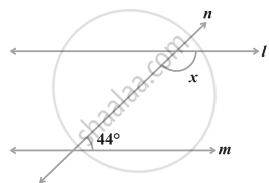Exercise 6.2 | Q 7 | Page 57

Two adjacent angles are equal. Is it necessary that each of these angles will be a right angle? Justify your answer.

Exercise 6.2 | Q 8 | Page 57

If one of the angles formed by two intersecting lines is a right angle, what can you say about the other three angles? Give reason for your answer.

Exercise 6.2 | Q 9 | Page 57

In figure, which of the two lines are parallel and why?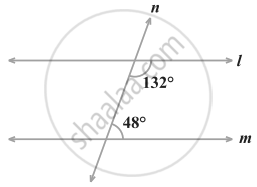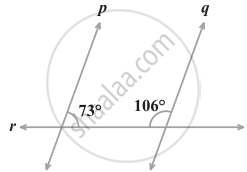Exercise 6.2 | Q 10 | Page 57

Two lines l and m are perpendicular to the same line n. Are l and m perpendicular to each other? Give reason for your answer.

Exercise 6.3 [Pages 58 - 60]

### NCERT solutions for Mathematics Exemplar Class 9 Chapter 6 Lines & Angles Exercise 6.3 [Pages 58 - 60]

Exercise 6.3 | Q 1 | Page 58

In the figure, OD is the bisector of ∠AOC, OE is the bisector of ∠BOC and OD ⊥ OE. Show that the points A, O and B are collinear.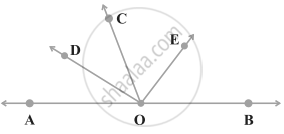Exercise 6.3 | Q 2 | Page 58

In the figure, ∠1 = 60° and ∠6 = 120°. Show that the lines m and n are parallel.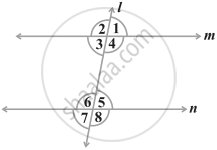Exercise 6.3 | Q 3 | Page 58

AP and BQ are the bisectors of the two alternate interior angles formed by the intersection of a transversal t with parallel lines l and m (figure). Show that AP || BQ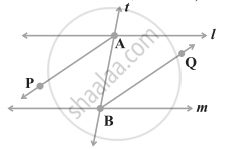Exercise 6.3 | Q 4 | Page 59

In the given figure, bisectors AP and BQ of the alternate interior angles are parallel, then show that l ||m.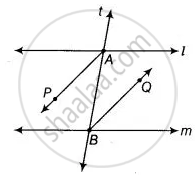Exercise 6.3 | Q 5 | Page 59

In the figure, BA || ED and BC || EF. Show that ∠ABC = ∠DEF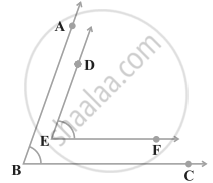Exercise 6.3 | Q 6 | Page 59

In the figure, BA || ED and BC || EF. Show that ∠ABC + ∠DEF = 180°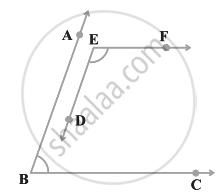Exercise 6.3 | Q 7 | Page 59

In the figure, DE || QR and AP and BP are bisectors of ∠EAB and ∠RBA, respectively. Find ∠APB.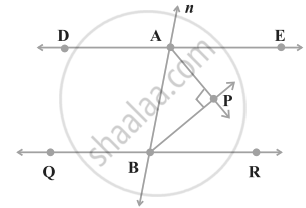Exercise 6.3 | Q 8 | Page 60

The angles of a triangle are in the ratio 2:3:4. Find the angles of the triangle.

Exercise 6.3 | Q 9 | Page 60

A triangle ABC is right-angled at A. L is a point on BC such that AL ⊥ BC. Prove that ∠BAL = ∠ACB.

Exercise 6.3 | Q 10 | Page 60

Two lines are respectively perpendicular to two parallel lines. Show that they are parallel to each other.

Exercise 6.4 [Pages 61 - 62]

### NCERT solutions for Mathematics Exemplar Class 9 Chapter 6 Lines & Angles Exercise 6.4 [Pages 61 - 62]

Exercise 6.4 | Q 1 | Page 61

If two lines intersect, prove that the vertically opposite angles are equal.

Exercise 6.4 | Q 2 | Page 61

Bisectors of interior ∠B and exterior ∠ACD of an ∆ABC intersect at the point T. Prove that ∠BTC = 1/2∠BAC

Exercise 6.4 | Q 3 | Page 61

A transversal intersects two parallel lines. Prove that the bisectors of any pair of corresponding angles so formed are parallel.

Exercise 6.4 | Q 4 | Page 62

Prove that through a given point, we can draw only one perpendicular to a given line.

Exercise 6.4 | Q 5 | Page 62

Prove that two lines that are respectively perpendicular to two intersecting lines intersect each other.

Exercise 6.4 | Q 6 | Page 62

Prove that a triangle must have atleast two acute angles.

Exercise 6.4 | Q 7 | Page 62

In the figure, ∠Q > ∠R, PA is the bisector of ∠QPR and PM ⊥ QR. Prove that ∠APM = 1/2 (∠Q – ∠R).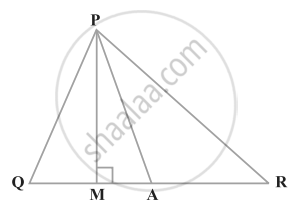## Chapter 6: Lines & Angles

Exercise 6.1Exercise 6.2Exercise 6.3Exercise 6.4## NCERT solutions for Mathematics Exemplar Class 9 chapter 6 - Lines & Angles

NCERT solutions for Mathematics Exemplar Class 9 chapter 6 (Lines & Angles) include all questions with solution and detail explanation. This will clear students doubts about any question and improve application skills while preparing for board exams. The detailed, step-by-step solutions will help you understand the concepts better and clear your confusions, if any. Shaalaa.com has the CBSE Mathematics Exemplar Class 9 solutions in a manner that help students grasp basic concepts better and faster.

Further, we at Shaalaa.com provide such solutions so that students can prepare for written exams. NCERT textbook solutions can be a core help for self-study and acts as a perfect self-help guidance for students.

Concepts covered in Mathematics Exemplar Class 9 chapter 6 Lines & Angles are Intersecting Lines and Non-intersecting Lines, Introduction to Lines and Angles, Basic Terms and Definitions, Parallel Lines, Pairs of Angles, Parallel Lines and a Transversal, Lines Parallel to the Same Line, Angle Sum Property of a Triangle.

Using NCERT Class 9 solutions Lines & Angles exercise by students are an easy way to prepare for the exams, as they involve solutions arranged chapter-wise also page wise. The questions involved in NCERT Solutions are important questions that can be asked in the final exam. Maximum students of CBSE Class 9 prefer NCERT Textbook Solutions to score more in exam.

Get the free view of chapter 6 Lines & Angles Class 9 extra questions for Mathematics Exemplar Class 9 and can use Shaalaa.com to keep it handy for your exam preparation